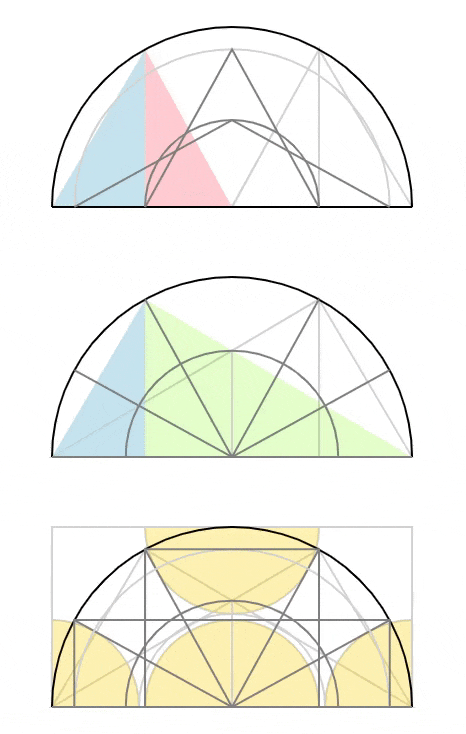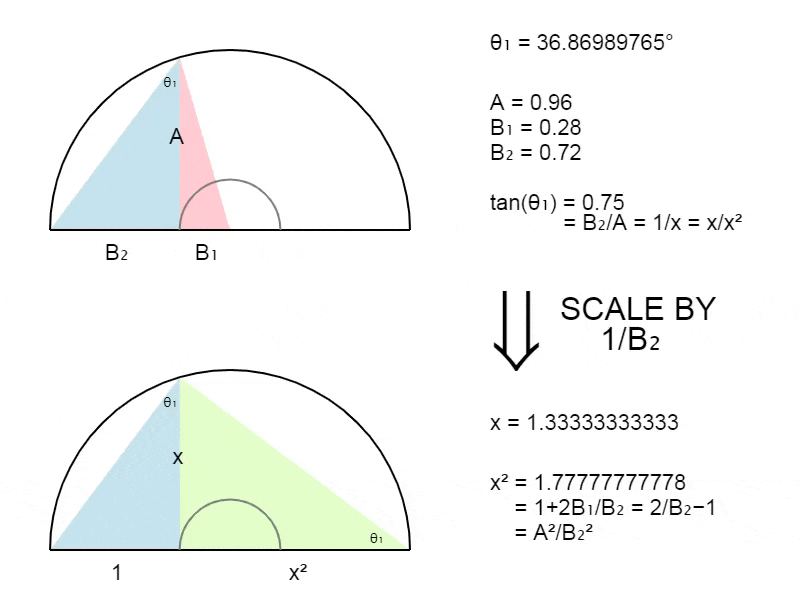Some interesting things regarding what I call the cosmic equation. This equation has facinatied me for years and seems to be the root of geometry.

cosmic identity
cosmic numbers
cosmic triangle
cosmic angles

We can link it with Pythagoras, trigonometry and the trigonometric functions, metallic means and many other mathematical

It is assumed that (x) is always ≥ 1 and the (1/x) is always ≤ 1.

## The Cosmic EquationLinking the 'cosmic' equation with Pythagoras.Linking the 'cosmic' equation with trigonometry and the trigonometric functions.Simplifying the tan relationship.more stuff.More stuff.## The Triangles

The relationship between the red and blue triangles.The relationship between the red and blue triangles.Animated example.Visualising the triangles using the cosmic equation.Substituting x₁ (from above) into the green triangle.## The Roots

Scale by 1/x₁ and substitute cos.Scale by x₁ and substitute cos.Animated example.To be continued..(last updated : 31/01/20)